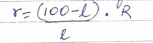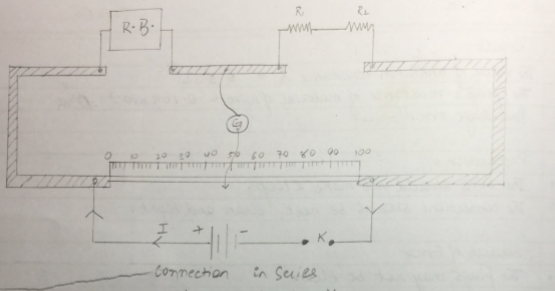To verify the laws of combination (series) of resistance using a meter bridge (R=R1 + R2 )

Aim : To verify the laws of combination (series) of resistance using a meter bridge (R=R1 + R2 )

Apparatus : a meter bridge , a leclanche cell (battery eliminator ) , a galvanometer , a resistance box , a jockey , two resistance wire or two resistance coils , set square , connecting wires .

Theory

1. The resistance (r) of a wire or coil is given byWhere R is the resistance from resistance box in the left gap and l is the length of the meter bridge wire from zero end upto balance point .

2. When two resistance r1 & r2 are connected in series , then their combined resistance

R3=R1+R2

Procedure

1. Mark the two resistance coil as r1 and r2 .
2. To find the r1 and r2 proceed same way as in experiment 1.

(if r1 and r2 are not known )

1. Connect the two coils r1 and r2 in series as shown circuit diagram in the right gap of meter bridge and find the resistance of this combination. Take at least three sets of observation.
2. Record your observation.

Result

Within limits of experimental error , experimental and theoretical values of R  are same . Hence law of resistance in series is verified .

Precaution

1. The key should be inserted only while taking observation .
2. Connections should be neat and tight .

Sources of error

1. The key in resistance box may be loose .
2. The galvanometer may not show opposite deflection .

Circuit diagramObservation table

 Resistance in R.B. (ohm) Balance l (cm) (100-l) (cm) [(100-l)*R]/l Mean r1 (cm) 0.5 24 76 1.583 1.616 1 38 62 1.631 2 55 45 1.636
 Resistance in R.B. (ohm) Balance l (cm) (100-l) (cm) [(100-l)*R]/l Mean r2 (cm) 0.5 33 67 1.015 1.015 1 50 50 1 2 66 34 1.030
 Resistance in R.B. (ohm) Balance l (cm) (100-l) (cm) [(100-l)*R]/l Mean rs (cm) 0.5 16 84 2.625 2.615 1 28 72 2.571 2 43 57 2.651

Calculation

R1 + R2 = 1.616+1.015 = 2.631 ohm
Rs = 2.615 ohm

Therefore, experimental error = [(2.615-2.631)/2.615]*100 = 0.6%

Viva question

How does resistance change in series combination ?

Ans. Resistance increase in series combination

Why does resistance increase in series combination ?

Ans. Effective length of resistor increases . As R*l , resistance increases .

What is resistance ?

Ans. it is the opposition offered by the material of two wire to flow of electric current .

What is the cause of resistance ?

Ans. it is the opposition offered by the material of wire to flow of electric current .

What is the effect of temperature In the resistance of conductor ?

Resistance of all conductor increases with increase in temperature .

You can also get Class XII Practicals on BiologyPhysics, and Physical Education.

15 Replies to “To verify the laws of combination (series) of resistance using a meter bridge (R=R1 + R2 )”

1.Shiny Sahara says:

I really loved it…… Thanks a lot for giving values here… 😊😇💞

1.Shivani says:

Thanku this help me a lottt for a clear verification

1.Raman Sharma says:

Yes

2.sneha dash says:

thanku so much

3.Sarfaraj Mallick says:

Thanks for helping to given this data.

4.Bipin Kumar says:

I want help me sir

5.Krish says:

How mean resistance come?

6.Nevedya says:

Thank you 😀

7.Shreemayee saswatika says:

It’s really good. Thank u sooo much 😊 😊 😊 😊

8.Pragyan says:

Thank you for giving values

9.ram says:

very nice thanku

10.Gaurav says:

Ty so much sir/ma’am
This is such good
I loved this..

11.Trilochan says:

Thanks a lot

12.Prachi Srivastava says:

Please give me parallel observation also… And thanks for this reading

13.Anjali Maurya says:

Thank u…